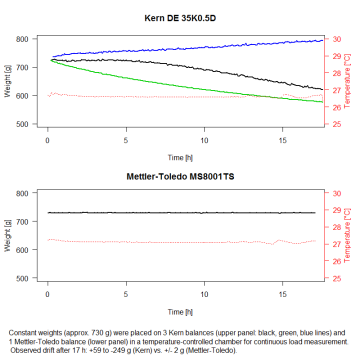# CR1 script to log balance measurements with a campbell scientific data logger

here’s a script to record time-series of balance measurements (weight data) using a Campbell Scientific CR1000 datalogger and a balance with an RS232 serial interface.
we have been using two dedicated scripts which vary slightly to work with two different balance models, the Mettler-Toledo MS8001TS (polled mode; should work with all current Mettler-Toledo balances based on MT-SICS) and the Kern DE35K0.5D1 (set to continuous output).

if you just want to have a look at the code, the CR1 scripts are included in text form at the end of this post.

1note that, as it turns out, the strain gauge loadcell measuring system of the Kern DE35K0.5D balance makes it unsuitable for longer term measurements. to get an idea of the drift effect resulting from a continuous load on these balances, here’s a figure from a test run with constant weights:CR1 script for one Mettler-Toledo MS8001TS balance
```'Log weight output from a Mettler-Toledo MT8001TS balance via Serial interface (RS232) 'On the balance in Settings > General configuration > Devices and connectivity, set RS232 to "PC-Direct".   'Declare Variables and Units Public Datastream As String * 50 Public ID As String *2 Public Status As String *2 Public Weight Public Unit As String *2 Public Batt Public PTemp   'Define Data Table DataTable(Weights,True,-1)) 'Set interval for recording data to 5 min DataInterval(0,5,min,10) Sample(1,Weight,IEEE4) Sample(1,Unit,String) Sample(1,Batt,IEEE4) Sample(1,PTemp,IEEE4) EndTable   BeginProg   'Open serial connection to balance SerialOpen(Com1,9600,3,0,1000)   'Start a reading every 5 seconds Scan(5,sec,3,0) 'Request weight value immediately (i.e. non-stable value) SerialOut(Com1,"SI"&CHR(13)&CHR(10),0,1,0) Delay(0,100,mSec) SerialIn(Datastream,Com1,100,10,50)   'Output example = "S D WeightValue Unit" ' characters: 1-2.1. 10 .1-X ID = Trim(Left(Datastream,2)) Status = Trim(Mid(Datastream,3,1)) Weight = Trim(Mid(Datastream,4,12)) ' remove 4 characters from beginning Unit = Trim(Mid(Datastream,15,10))   Battery(Batt) 'Datalogger Battery Voltage PanelTemp(PTemp,_50Hz) 'Wiring Panel Temperature   CallTable Weights NextScan EndProg```

CR1 script for one Kern DE350K0.5D balance
```'Log weight output from a Kern DE350K0.5D balance via Serial interface (RS232) 'On the balance, set output mode to "Auto PC".   'Declare Variables and Units Public Datastream As String * 50 Public Readfrom As Long Public Plusminus As String *1 Public Weight Public Unit As String *2 Public Batt Public PTemp   'Define Data Table DataTable(Weights,True,-1)) 'Set interval for recording data to 5 min DataInterval(0,5,min,10) Sample(1,Weight,IEEE4) Sample(1,Unit,String) Sample(1,Batt,IEEE4) Sample(1,PTemp,IEEE4) EndTable   BeginProg   'Open serial connection to balance SerialOpen(Com1,9600,3,0,1000)   'Start a reading every 5 seconds Scan(5,sec,3,0)   'Read continuous output(non-stable value) SerialIn(Datastream,Com1,100,0,70)   'Find where new line starts (line-feed character, ASCI dec.10) Readfrom = InStr(1,Datastream,CHR(10),2) Weight = Trim(Mid(Datastream,Readfrom+3,14)) 'Extract minus sign (separated by spaces), apply if the value is negative Plusminus = Mid(Datastream,Readfrom+2,1) If Plusminus = "-" Then Weight = Weight * -1 EndIf Unit = Trim(Mid(Datastream,Readfrom-4,2))   Battery(Batt) 'Datalogger Battery Voltage PanelTemp(PTemp,_50Hz) 'Wiring Panel Temperature   CallTable Weights NextScan EndProg```

CR1 script for four Kern DE350K0.5D balances
```'This is the Campbell CR1000 code for logging the weight with 4 Kern DE 35K0.5D balances 'On the balance, set output mode to "Auto PC".   'Declare Variables and Units Public Datastream1 As String * 50, Datastream2 As String * 50, Datastream3 As String * 50, Datastream4 As String * 50 Public Readfrom1 As Long, Readfrom2 As Long, Readfrom3 As Long, Readfrom4 As Long Public Plusminus1 As String *1, Plusminus2 As String *1, Plusminus3 As String *1, Plusminus4 As String *1 Public Weight1, Weight2, Weight3, Weight4 Public Unit1 As String *2, Unit2 As String *2, Unit3 As String *2, Unit4 As String *2   Public Batt Public PTemp   'Define Data Table DataTable (Weights,True,-1)) 'Set interval for recording data to 5 min DataInterval (0,5,min,10) Sample (1,Weight1,IEEE4) Sample (1,Unit1,String) Sample (1,Weight2,IEEE4) Sample (1,Unit2,String) Sample (1,Weight3,IEEE4) Sample (1,Unit3,String) Sample (1,Weight4,IEEE4) Sample (1,Unit4,String) Sample (1,Batt,IEEE4) Sample (1,PTemp,IEEE4) EndTable   BeginProg   'Open serial connection to balances SerialOpen (Com1,9600,3,0,1000) SerialOpen (Com2,9600,3,0,1000) SerialOpen (Com3,9600,3,0,1000) SerialOpen (Com4,9600,3,0,1000)   'Start a reading every 5 seconds Scan (5,sec,3,0)   'Read continuous output (non-stable value) SerialIn(Datastream1,Com1,100,0,70) SerialIn(Datastream2,Com2,100,0,70) SerialIn(Datastream3,Com3,100,0,70) SerialIn(Datastream4,Com4,100,0,70)   'Balance 1 'Find where new line starts (line-feed character, ASCI dec.10) Readfrom1 = InStr(1,Datastream1,CHR(10),2) Weight1 = Trim(Mid(Datastream1,Readfrom1+3,14)) 'Extract minus sign (separated by spaces), apply if the value is negative Plusminus1 = Mid(Datastream1,Readfrom1+2,1) If Plusminus1 = "-" Then Weight1 = Weight1 * -1 EndIf Unit1 = Trim(Mid(Datastream1,Readfrom1-4,2))   'Balance 2 'Find where new line starts (line-feed character, ASCI dec.10) Readfrom2 = InStr(1,Datastream2,CHR(10),2) Weight2 = Trim(Mid(Datastream2,Readfrom2+3,14)) 'Extract minus sign (separated by spaces), apply if the value is negative Plusminus2 = Mid(Datastream2,Readfrom2+2,1) If Plusminus2 = "-" Then Weight2 = Weight2 * -1 EndIf Unit2 = Trim(Mid(Datastream2,Readfrom2-4,2))   'Balance 3 'Find where new line starts (line-feed character, ASCI dec.10) Readfrom3 = InStr(1,Datastream3,CHR(10),2) Weight3 = Trim(Mid(Datastream3,Readfrom3+3,14)) 'Extract minus sign (separated by spaces), apply if the value is negative Plusminus3 = Mid(Datastream3,Readfrom3+2,1) If Plusminus3 = "-" Then Weight3 = Weight3 * -1 EndIf Unit3 = Trim(Mid(Datastream3,Readfrom3-4,2))   'Balance 4 'Find where new line starts (line-feed character, ASCI dec.10) Readfrom4 = InStr(1,Datastream4,CHR(10),2) Weight4 = Trim(Mid(Datastream4,Readfrom4+3,14)) 'Extract minus sign (separated by spaces), apply if the value is negative Plusminus4 = Mid(Datastream4,Readfrom4+2,1) If Plusminus4 = "-" Then Weight4 = Weight4 * -1 EndIf Unit4 = Trim(Mid(Datastream4,Readfrom4-4,2))   Battery (Batt) 'Default Datalogger Battery Voltage measurement PanelTemp (PTemp,_50Hz) 'Wiring Panel Temperature measurement   CallTable Weights NextScan EndProg```

This site uses Akismet to reduce spam. Learn how your comment data is processed.